Question

# A certain ideal gas has atomic mass number 622. A flask of volume 0.0277 m 3...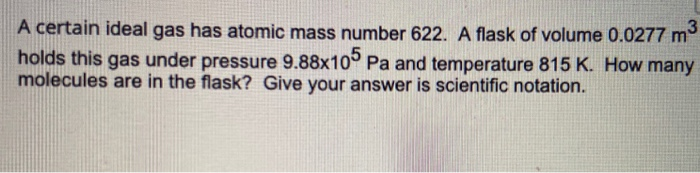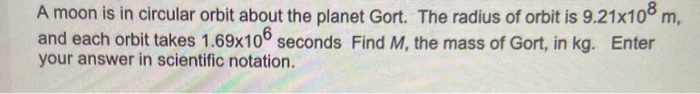A certain ideal gas has atomic mass number 622. A flask of volume 0.0277 m 3 holds this gas under pressure 9.88x105 Pa and temperature 815 K. How many molecules are in the flask? Give your answer is scientific notation.
A moon is in circular orbit about the planet Gort. The radius of orbit is 9.21x108 m, and each orbit takes 1.69x106 seconds Find M, the mass of Gort, in kg. Enter your answer in scientific notation.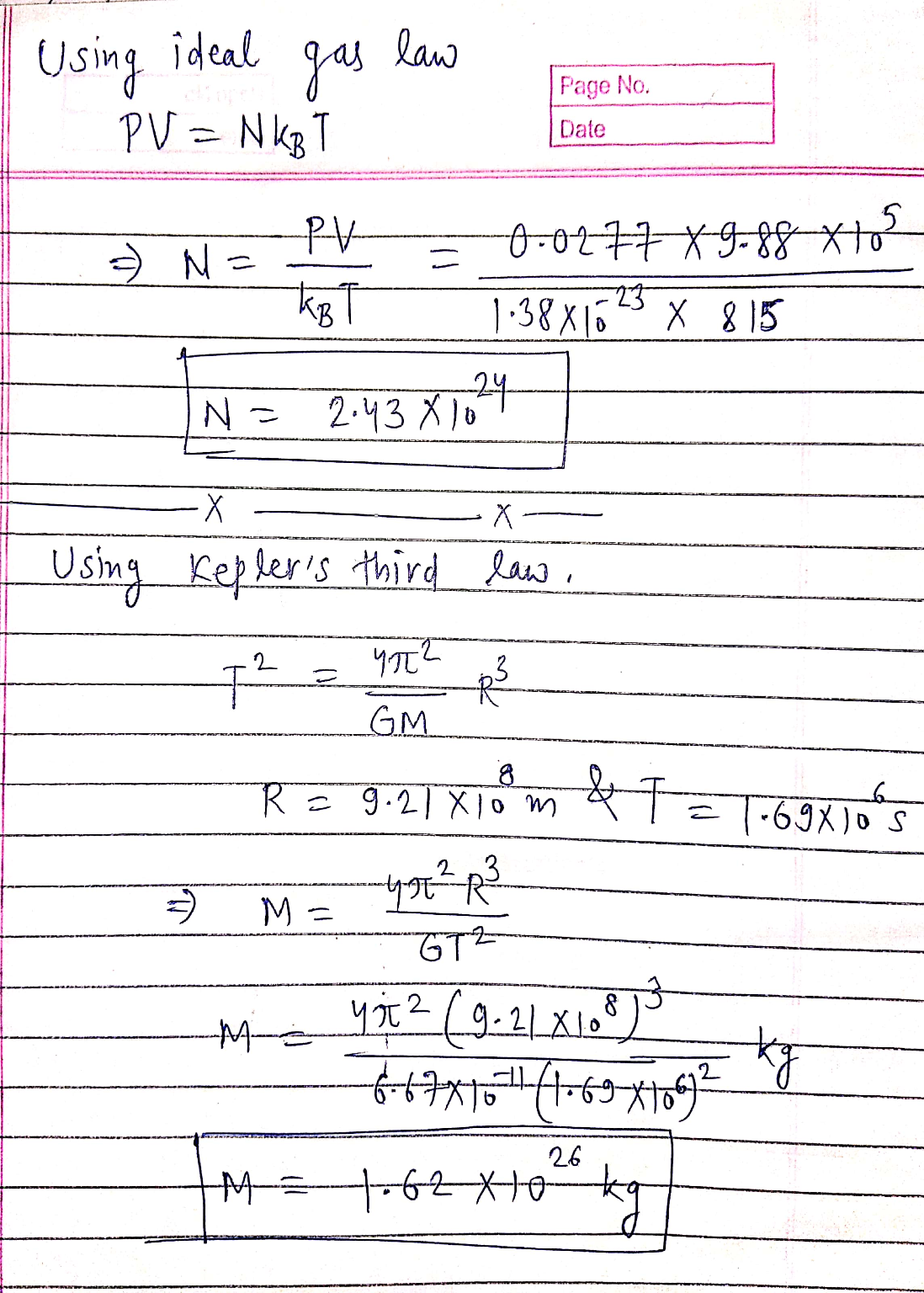#### Earn Coins

Coins can be redeemed for fabulous gifts.

Similar Homework Help Questions
• ### A flask holds 8.75 kg of a diaatomic ideal gas (mass number of the gas 82,8)....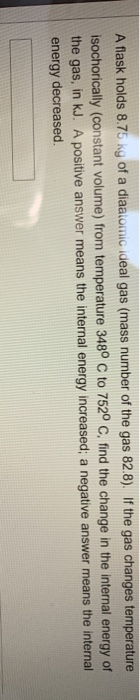A flask holds 8.75 kg of a diaatomic ideal gas (mass number of the gas 82,8). If the gas changes temperature isochorically (constant volume) from temperature 348° C to 752° C, find the change in the internal energy of the gas, in kJ. A positive answer means the internal energy increased, a negative answer means the internal energy decreased. A flask holds 5.11 kg of a monatomic ideal gas (mass number 283). If the gas changes temperature isobarically (constant pressure)...

• ### The spaceship Asimov is in circular orbit (radius 6.02x107 m) about the planet Terminus. If it...

The spaceship Asimov is in circular orbit (radius 6.02x107 m) about the planet Terminus. If it takes 5,004 seconds to orbit the planet, find the mass of the planet, in kg. Express your answer inscientific notation (using E for exponent).

• ### Question 3 1 pts A gas tank with a volume of 22.1 L contains 4.1 moles...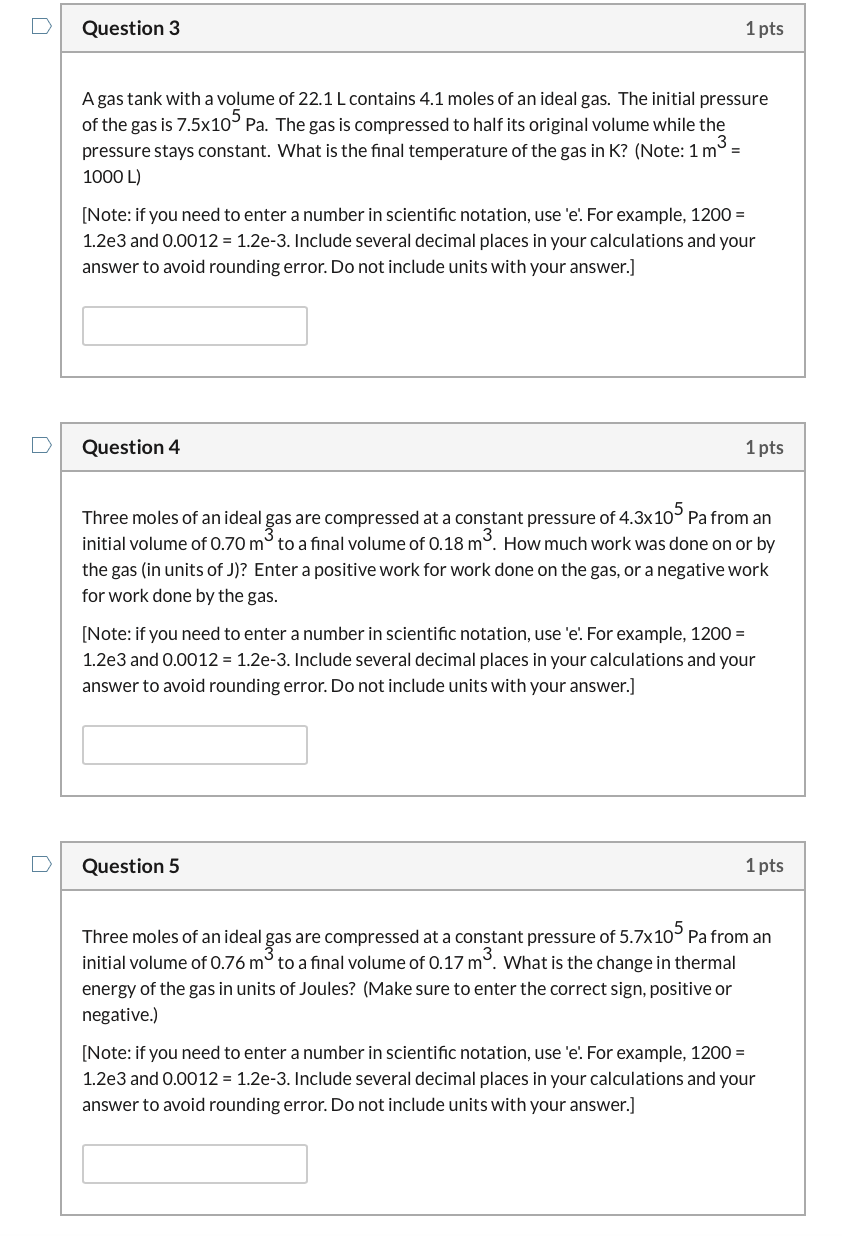Question 3 1 pts A gas tank with a volume of 22.1 L contains 4.1 moles of an ideal gas. The initial pressure of the gas is 7.5x10° Pa. The gas is compressed to half its original volume while the pressure stays constant. What is the final temperature of the gas in K? (Note: 1 mº = 1000 L) [Note: if you need to enter a number in scientific notation, use 'e. For example, 1200 = 1.2e3 and 0.0012 =...

• ### QUESTION 16 The Moon which has mass of about 7.35 1022 kilograms, and it orbits the...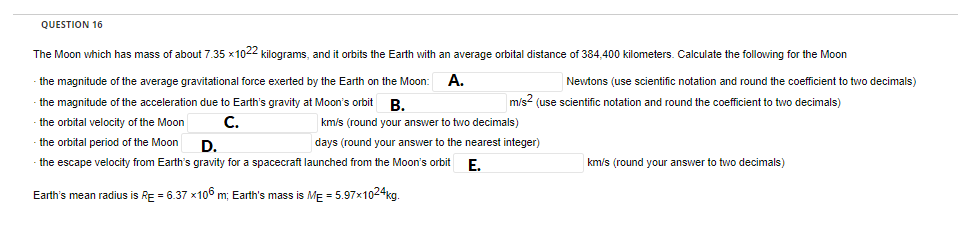QUESTION 16 The Moon which has mass of about 7.35 1022 kilograms, and it orbits the Earth with an average orbital distance of 384,400 kilometers. Calculate the following for the Moon the magnitude of the average gravitational force exerted by the Earth on the Moon: A. Newtons (use scientific notation and round the coefficient to two decimals) the magnitude of the acceleration due to Earth's gravity at Moon's orbit B. m/s (use scientific notation and round the coefficient to two...

• ### An asteroid of mass m is in a perfectly circular orbit of radius d about a...

An asteroid of mass m is in a perfectly circular orbit of radius d about a planet of mass M. You may assume that M>>m so that M is completely stationary. a. Determine an expression for the orbital period of the asteroid. (I already completed this step. Answer is T=2pi(r^3/2)/sqrt(GM) b. The asteroid is suddenly stopped in its tracks and allowed to fall freely towards the planet. Determine the time it takes to collide with the planet (assumed to have...

• ### Calculate molar volume of an ideal gas in m^3 at room temperature and pressure (or RTP)....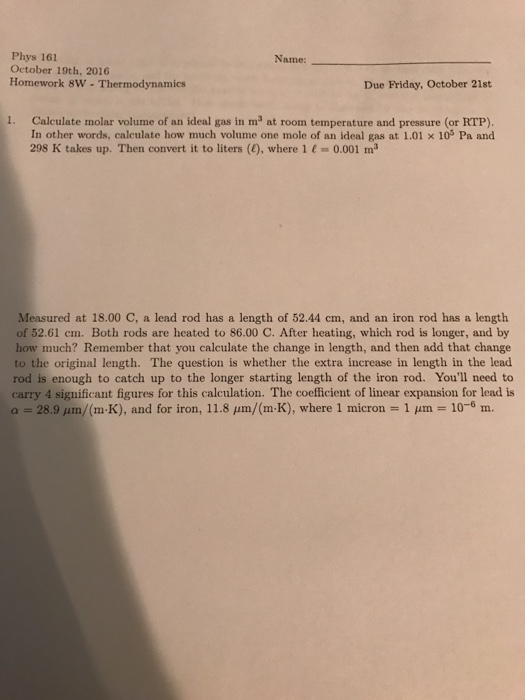Calculate molar volume of an ideal gas in m^3 at room temperature and pressure (or RTP). Id other words, calculate how much volume one mole of an ideal gas at 1.01 times 10^5 Pa and 298 K takes up. Then convert it to liters (l), where 1 t - 0.001 m^3 Measured at 18.00 C, a lead rod has a length of 52.44 cm, and an iron rod has a length of 52.61 cm. Both rods are heated to 86.0...

• ### There are 5.00x10 26 atoms/m 3 in a container of argon gas (atomic mass number 40)....

There are 5.00x10 26 atoms/m 3 in a container of argon gas (atomic mass number 40). The pressure in the container when the atoms have a rms speed of 350 m/s is: A. 4.53x10 4 N/m 2 B. 1.01x10 4 N/m 2 C. 1.01x10 5 N/m 2 D. 1.36x10 5 N/m 2 E. None of the above.

• ### See diagram 4. 22.8 moles of a diatomic ideal gas undergo three steps: A to B...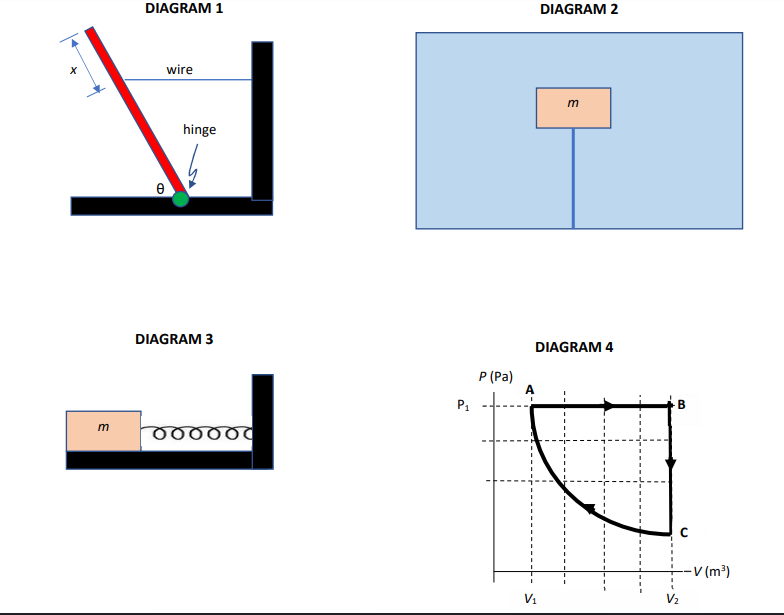See diagram 4. 22.8 moles of a diatomic ideal gas undergo three steps: A to B is an isobaric (constant pressure P1 = 5.25x106 Pascal) expansion from volume V1 = 0.0248 m3 to V2 = 0.956 m3. B to C is isochoric (constant volume) C to A is isothermal (constant T). During the isobaric expansion from A to B: find Q, the heat transferred, in Joules. Give your answer in scientific notation. NOTE: A positive sign means heat has been...

• ### b. ii) Use particle mass and k. A container holds 0.031 moles of monatomic Argon (atomic...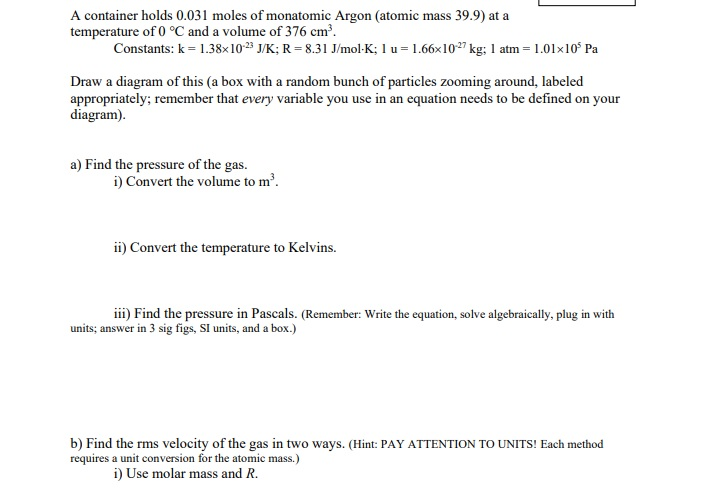b. ii) Use particle mass and k. A container holds 0.031 moles of monatomic Argon (atomic mass 39.9) at a temperature of 0 °C and a volume of 376 cm? Constants: k = 1.38x10-2 J/K; R=8.31 J/mol-K; l u = 1.66x10-27 kg: 1 atm = 1.01x10 Pa Draw a diagram of this (a box with a random bunch of particles zooming around, labeled appropriately; remember that every variable you use in an equation needs to be defined on your diagram)....

• ### A physics lecture room has a volume of 278 m^3 . Part A For a pressure...

A physics lecture room has a volume of 278 m^3 . Part A For a pressure of 1.00 atm and a temperature of 27.0 ∘C, use the ideal-gas law to estimate the number of air molecules in the room. Assume all the air is N2. Express your answer to three significant figures and include the appropriate units. N = ?    molecules Part B Calculate the particle density-that is, the number of N2 molecules per cubic centimeter. Express your answer to...